## Yff PointsLet points,, andbe marked off some fixed distancealong each of the sides,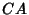, and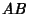. Then the lines,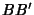, andconcur in a pointknown as the first Yff point if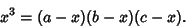(1)

This equation has a single real root, which can by obtained by solving the Cubic Equation(2)

where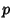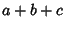(3)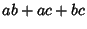(4)(5)

The Isotomic Conjugate Pointis called the second Yff point. The Triangle Center Functions of the first and second points are given by(6)

and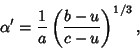(7)

respectively. Analogous to the inequalityfor the Brocard Angle,holds for the Yff points, with equality in the case of an Equilateral Triangle. Analogous to(8)

for, 2, 3, the Yff points satisfy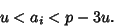(9)

Yff (1963) gives a number of other interesting properties. The lineis Perpendicular to the line containing the Incenter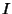and Circumcenter, and its length is given by(10)

where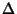is the Area of the Triangle.

Yff, P. An Analog of the Brocard Points.'' Amer. Math. Monthly 70, 495-501, 1963.